# Chapter 7 Frequencies

## 7.1 Binomial Test

### 7.1.1 Example

An example from Hays (1974, pp. 190-192):

“Think of a hypothetical study of this question: ‘If a human is subjected to a stimulus below his threshold of conscious awareness, can his behavior somehow still be influenced by the presence of the stimulus?’ The experimental task is as follows: the subject is seated in a room in front of a square screen divided into four equal parts. He is instructed that his task is to guess in which part of the screen a small, very faint, spot of light is thrown.”

Under the null hypothesis H0, the number of correct guesses is expected to be 1/4 of the trials N. The alternative hypothesis H1 is that the number of correct guesses is larger than 1/4 of the trials N.

The subject obtained 7 correct guesses T out of 10 trials N.

What is the p-value of this result under H0?

p = 0.25

N = 10

T = 7

### 7.1.2 Results Overview

Table 7.1: Result Overview Binomial Test
By Hand JASP SPSS SAS Minitab R
P 0.0035 0.0035 0.004 0.0035 0.004 0.0035

### 7.1.3 By Hand

Calculations by hand can be found in Hays, 1974, pp. 190-192.

Result: P = 0.0035

### 7.1.4 JASP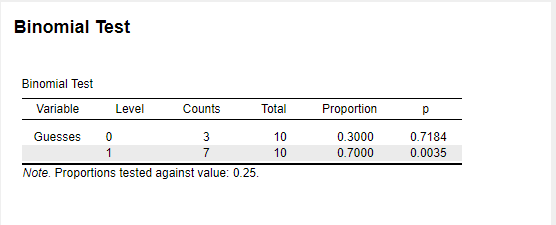Figure 7.1: JASP Output for Binomial Test

### 7.1.5 SPSS

DATASET NAME DataSet1 WINDOW=FRONT.
*Nonparametric Tests: One Sample.
NPTESTS
/ONESAMPLE TEST (Guesses) BINOMIAL(TESTVALUE=0.25 SUCCESSCATEGORICAL=FIRST SUCCESSCONTINUOUS=CUTPOINT(MIDPOINT))
/MISSING SCOPE=ANALYSIS USERMISSING=EXCLUDE
/CRITERIA ALPHA=0.05 CILEVEL=95.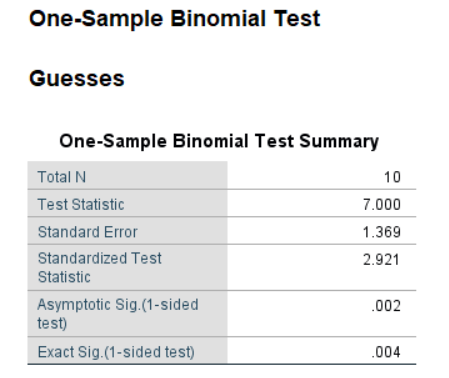Figure 7.2: SPSS Output for Binomial Test

### 7.1.6 SAS

PROC Freq data=WORK.IMPORT;
tables Guesses / binomial(p=.25 level=2);
exact binomial;
run;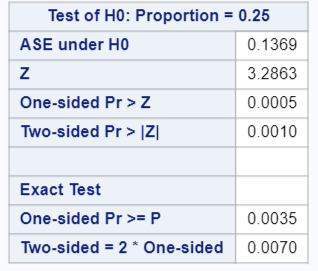Figure 7.3: SAS Output for Binomial Test

### 7.1.7 Minitab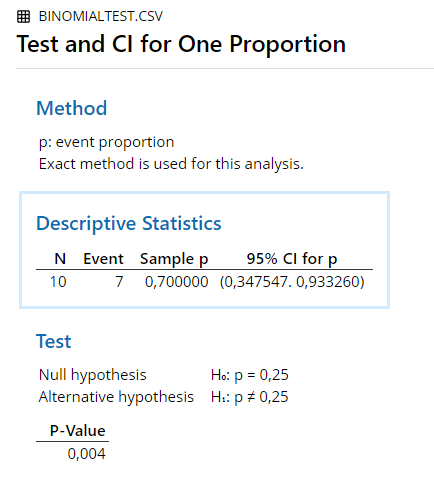Figure 7.4: Minitab Output for Binomial Test

### 7.1.8 R

binom.test(7, 10, p = 0.25, alternative = "greater")
##
## 	Exact binomial test
##
## data:  7 and 10
## number of successes = 7, number of trials = 10, p-value = 0.003506
## alternative hypothesis: true probability of success is greater than 0.25
## 95 percent confidence interval:
##  0.3933758 1.0000000
## sample estimates:
## probability of success
##                    0.7

### 7.1.9 Remarks

All differences in results between the software and hand calculation are due to rounding.

### 7.1.10 References

Hays, W. L. (1974). Statistics for the social sciences (2nd Ed.). New York, US: Holt, Rinehart and Winston, Inc.

## 7.2 Multinomial Test / Chi-square Goodness of Fit Test

### 7.2.1 Example

Think of colored marbles mixed together in a box, where the following probability distribution holds:

Table 7.2: Probability Distribution for Multinomial Test Example
Color p
Black 0.4
Red 0.3
White 0.3

Now suppose that 10 marbles were drawn at random and with replacement. The samples shows 2 black, 3 red, and 5 white.

Table 7.3: Sample for Multinomial Test Example
Color Count Expected
Black 2 4
Red 3 3
White 5 3

### 7.2.2 Results Overview

Table 7.4: Result Overview Multinomial Test
JASP SPSS SAS Minitab R
$$\chi ^2$$ 2.333 2.333 2.333 2.333 2.333

### 7.2.3 JASP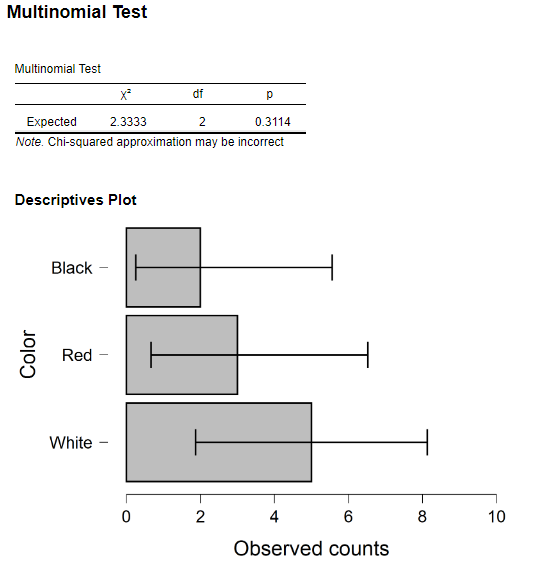Figure 7.5: JASP Output for Multinomial Test

### 7.2.4 SPSS

DATASET ACTIVATE DataSet1.
NPAR TESTS
/CHISQUARE=Numbered
/EXPECTED=4 3 3
/MISSING ANALYSIS.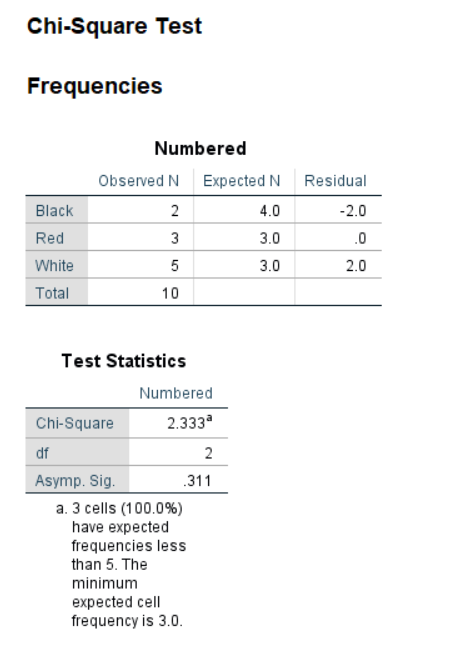Figure 7.6: SPSS Output for Multinomial Test

### 7.2.5 SAS

PROC FREQ DATA = chisquared;
TABLES Sex*Preference / chisq;
run;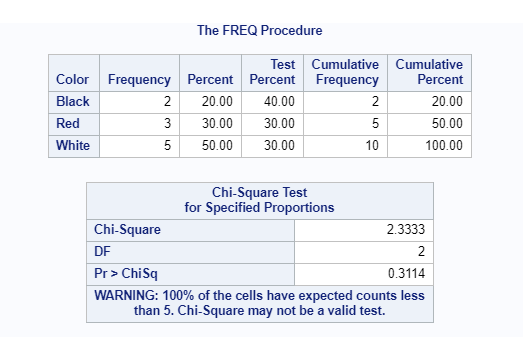Figure 7.7: SAS Output for Multinomial Test

### 7.2.6 Minitab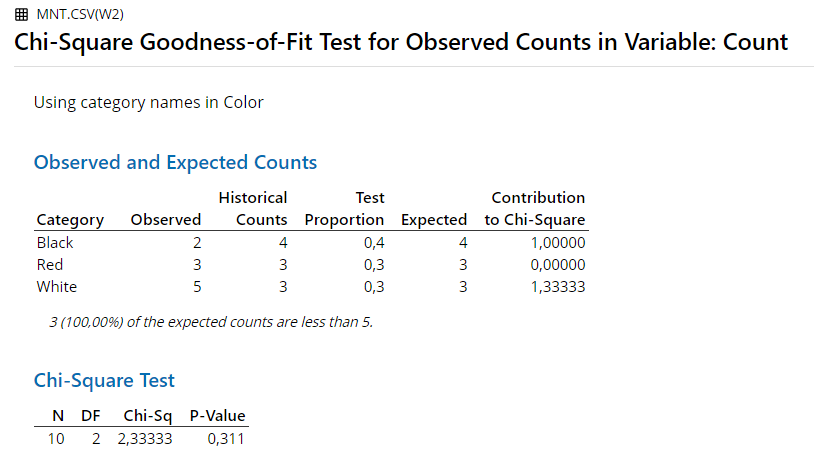Figure 7.8: Minitab Output for Multinomial Test

### 7.2.7 R

chisq.test(MNsample$Count, p = Pdist$p)
## Warning in chisq.test(MNsample$Count, p = Pdist$p): Chi-squared approximation
## may be incorrect
##
## 	Chi-squared test for given probabilities
##
## data:  MNsample\$Count
## X-squared = 2.3333, df = 2, p-value = 0.3114

### 7.2.8 Remarks

All differences in results between the software are due to rounding.

## 7.3 Chi-Squared-Test

An example from Hays (1974, pp. 728-731):

“For example, suppose that a random sample of 1– school children is drawn. Each child is classified in two ways: the first attribute is the sex of the child, with two possible categories: [Male, Female]. The second attribute […] is the stated preference of a child for two kinds of reading materials: [Fiction, Nonfiction]. […] The data might, for example, turn out to be:”

Table 7.5: Data for Chi-Squared-Test
Male Female
Fiction 19 32
Nonfiction 29 20

### 7.3.1 Results Overview

Table 7.6: Result Overview Chi-Squared-Test
By Hand JASP SPSS SAS Minitab R
$$\chi ^2$$ 4.83 4.8145 4.814 4.8145 4.814 4.8145

### 7.3.2 By Hand

Calculations by hand can be found in Hays, 1974, pp. 728-731.

Result: $$\chi ^2$$ = 4.83

Significant for $$\alpha$$ = .05 or less

### 7.3.3 JASP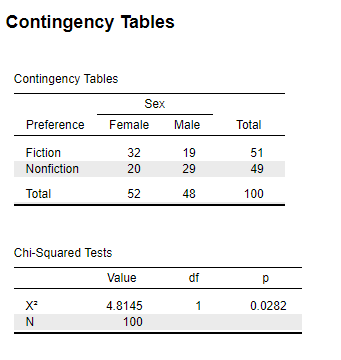Figure 7.9: JASP Output for Chi-Squared-Test

### 7.3.4 SPSS

CROSSTABS
/TABLES=Sex BY Preference
/FORMAT=AVALUE TABLES
/STATISTICS=CHISQ
/CELLS=COUNT
/COUNT ROUND CELL.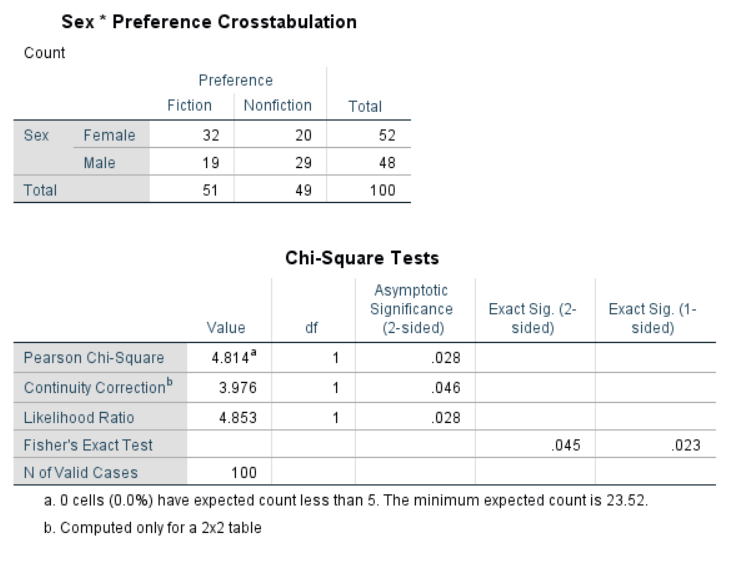Figure 7.10: SPSS Output for Chi-Squared-Test

### 7.3.5 SAS

PROC FREQ DATA = chisquared;
TABLES Sex*Preference / chisq;
run;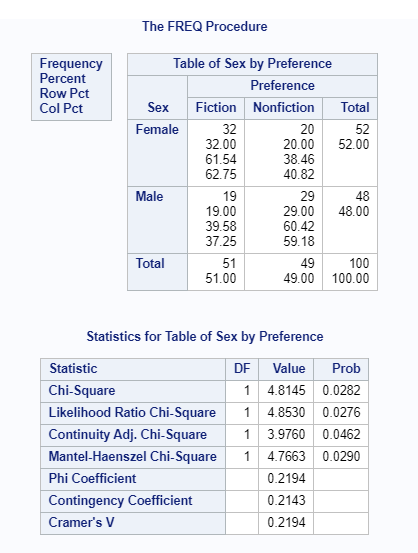Figure 7.11: SAS Output for Chi-Squared-Test

### 7.3.6 Minitab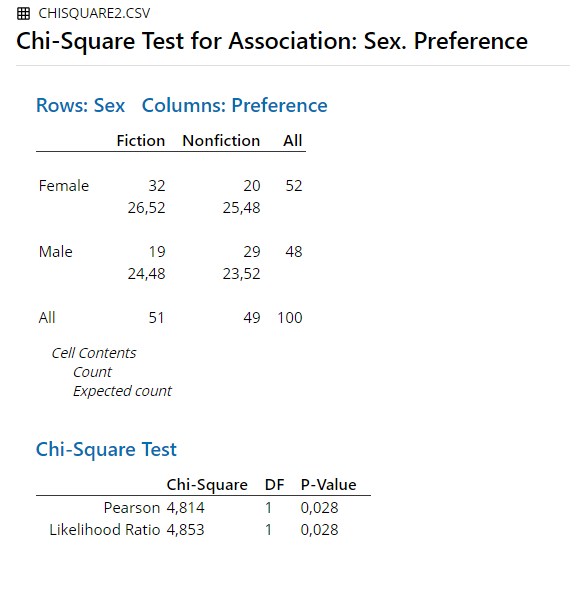Figure 7.12: Minitab Output for Chi-Squared-Test

### 7.3.7 R

chisq.test(chiSquare.data, correct = F)
##
## 	Pearson's Chi-squared test
##
## data:  chiSquare.data
## X-squared = 4.8145, df = 1, p-value = 0.02822

### 7.3.8 Remarks

All differences in results between the software and hand calculation are due to rounding.

### 7.3.9 References

Hays, W. L. (1974). Statistics for the social sciences (2nd Ed.). New York, US: Holt, Rinehart and Winston, Inc.